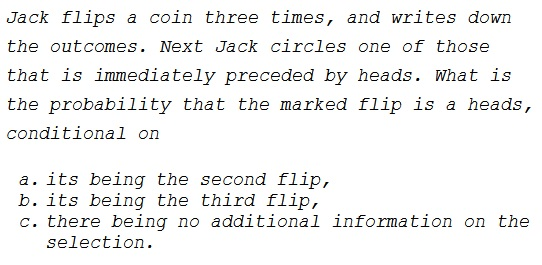# A Search for heads and Its Consequences - After Miller & Sanjurjo

### Problem### Solution, Question 1

There are four possible outcomes for the first two flips: $TT,$ $TH,$ $HT,$ $HH.$ Since Jack selects a flip immediately preceded by a heads, the first two sequences could not have led to his selection of the second flip. Two possibilities remain: $HT$ and $HH$ which are equiprobable. However, in the second case, Jack had a choice between selecting the second or the third flips. Thus the probability of selecting heads is split. Hence, the answer is $\displaystyle \frac{1}{3}.$

### Solution, Question 2

Since the third flip was selected, the second one is bound to be heads. Thus we have four possibilities: $THT,$ $THH,$ $HHT,$ $HHH.$ All equiprobable, so that the answer in this case is also $\displaystyle \frac{1}{2}.$

### Solution, Question 3

We do not know what was Jack's selection but we do know that there a heads came up in the first flip or the second (or both). Thus we have six possible sequences (i.e., excluding $TTT$ and $TTH):$ $HTT,$ $HTH,$ $THT,$ $THH,$ $HHT,$ $HHH.$ Each of these has the probability of $\displaystyle \frac{1}{6}$ of occurring. In the first four Jack has no choice and is bound to selecting a unique one. His election is a heads in just one case, $THH,$ i.e., with the probability of $\displaystyle \frac{1}{6}.$ Of the remaining two cases, where Jack has a choice to make, a heads would be selected in three out of four possibilities: $H\textbf{H}T,$ $HH\textbf{T},$ $H\textbf{H}H,$ $HH\textbf{H}.$ Thus, the probability of a heads flip being selected is $\displaystyle \frac{1}{2}\cdot\frac{3}{6}=\frac{1}{4}.$

Combining the two cases, a heads would be selected with the probability of $\displaystyle \frac{1}{6}+\frac{1}{4}=\frac{5}{12}.$

A shorter way is to combine the answers to the first two questions, since it is equally likely to be the first or second flip, so $\displaystyle \frac{1}{2}\cdot\frac{1}{2}+\frac{1}{2}\cdot\frac{1}{3}=\frac{5}{12}.$

### Remark

Above we assumed that Jack - when given a choice - makes his selection at random. In case this is not true, e.g., if Jack is mildly autistic and always chooses the first of the two possibilities or always a heads when he can, to start with, the answers to the questions would have come differently.

### Acknowledgment

This is a paraphrase of a problem that was kindly communicated to me by Joshua B. Miller. Joshua mentions that the idea of the problem emerged from the joint work with Adam Sanjurjo on the hot-hand fallacy.

• Miller, Joshua B. and Sanjurjo, Adam, A Bridge from Monty Hall to the Hot Hand: Restricted Choice, Selection Bias, and Empirical Practice (October 27, 2017). IGIER Working Paper. Available at SSRN and dx.doi.org.

In preparing this page I was selflessly helped by Alejandro Rodríguez, Thamizh Kudimagan and Zhuo Xi.